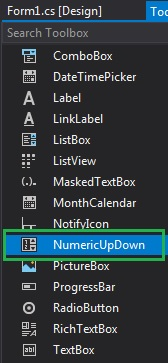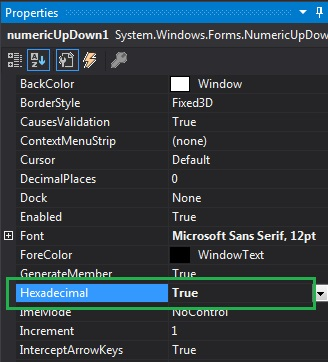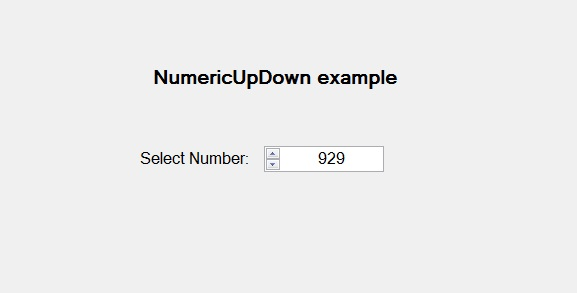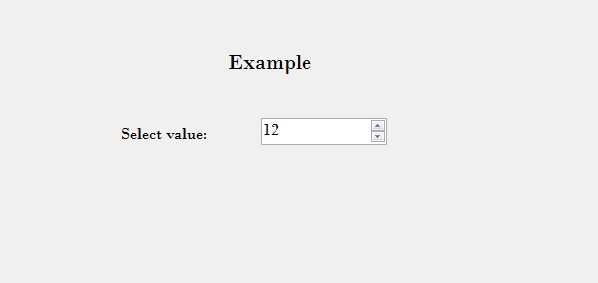# How to display Hexadecimal Values in NumericUpDown in C#?

In Windows Forms, NumericUpDown control is used to provide a Windows spin box or an up-down control which displays the numeric values. Or in other words, NumericUpDown control provides an interface which moves using up and down arrow and holds some pre-defined numeric value. In NumericUpDown control, you can display values in hexadecimal format in the up-down control using Hexadecimal Property.
If the value of this property is set to true, then the up-down control displays the values in hexadecimal format. And if the value of this property is set to false, then the up-down control does not display the values in hexadecimal format. The default value of this property is false. You can set this property in two different ways:

1. Design-Time: It is the easiest way to set the hexadecimal values in the NumericUpDown as shown in the following steps:

• Step 1: Create a windows form as shown in the below image:
Visual Studio -> File -> New -> Project -> WindowsFormApp• Step 2: Next, drag and drop the NumericUpDown control from the toolbox on the form as shown in the below image:• Step 3: After drag and drop you will go to the properties of the NumericUpDown and set the hexadecimal values in the NumericUpDown as shown in the below image:Output:2. Run-Time: It is a little bit trickier than the above method. In this method, you can set in the hexadecimal format in the NumericUpDown control programmatically with the help of given syntax:

`public bool Hexadecimal { get; set; }`

The value of this property is of System.Boolean type, either true or false. The following steps show how to set the hexadecimal values in the NumericUpDown dynamically:

• Step 1: Create a NumericUpDown using the NumericUpDown() constructor is provided by the NumericUpDown class.
```// Creating a NumericUpDown
NumericUpDown n = new NumericUpDown();
```
• Step 2: After creating NumericUpDown, set the Hexadecimal property of the NumericUpDown provided by the NumericUpDown class.
```// Setting the Hexadecimal values
```
• Step 3: And last add this NumericUpDown control to the form using the following statement:
```// Adding NumericUpDown control on the form
```

Example:

 `using` `System; ` `using` `System.Collections.Generic; ` `using` `System.ComponentModel; ` `using` `System.Data; ` `using` `System.Drawing; ` `using` `System.Linq; ` `using` `System.Text; ` `using` `System.Threading.Tasks; ` `using` `System.Windows.Forms; ` ` `  `namespace` `WindowsFormsApp44 { ` ` `  `public` `partial` `class` `Form1 : Form { ` ` `  `    ``public` `Form1() ` `    ``{ ` `        ``InitializeComponent(); ` `    ``} ` ` `  `    ``private` `void` `Form1_Load(``object` `sender, EventArgs e) ` `    ``{ ` ` `  `        ``// Creating and setting the ` `        ``// properties of the labels ` `        ``Label l1 = ``new` `Label(); ` `        ``l1.Location = ``new` `Point(348, 61); ` `        ``l1.Size = ``new` `Size(215, 25); ` `        ``l1.Text = ``"Example"``; ` `        ``l1.Font = ``new` `Font(``"Bodoni MT"``, 16); ` `        ``this``.Controls.Add(l1); ` ` `  `        ``Label l2 = ``new` `Label(); ` `        ``l2.Location = ``new` `Point(242, 136); ` `        ``l2.Size = ``new` `Size(103, 20); ` `        ``l2.Text = ``"Select value:"``; ` `        ``l2.Font = ``new` `Font(``"Bodoni MT"``, 12); ` `        ``this``.Controls.Add(l2); ` ` `  `        ``// Creating and setting the ` `        ``// properties of NumericUpDown ` `        ``NumericUpDown n = ``new` `NumericUpDown(); ` `        ``n.Location = ``new` `Point(386, 130); ` `        ``n.Size = ``new` `Size(126, 26); ` `        ``n.Font = ``new` `Font(``"Bodoni MT"``, 12); ` `        ``n.Value = 18; ` `        ``n.Minimum = 18; ` `        ``n.Maximum = 30; ` `        ``n.Increment = 1; ` `        ``n.Hexadecimal = ``true``; ` ` `  `        ``// Adding this control ` `        ``// to the form ` `        ``this``.Controls.Add(n); ` `    ``} ` `} ` `} `

Output:Whether you're preparing for your first job interview or aiming to upskill in this ever-evolving tech landscape, GeeksforGeeks Courses are your key to success. We provide top-quality content at affordable prices, all geared towards accelerating your growth in a time-bound manner. Join the millions we've already empowered, and we're here to do the same for you. Don't miss out - check it out now!

Previous
Next